Ex 15.1

Chapter 15 Class 9 Probability (Deleted)
Serial order wise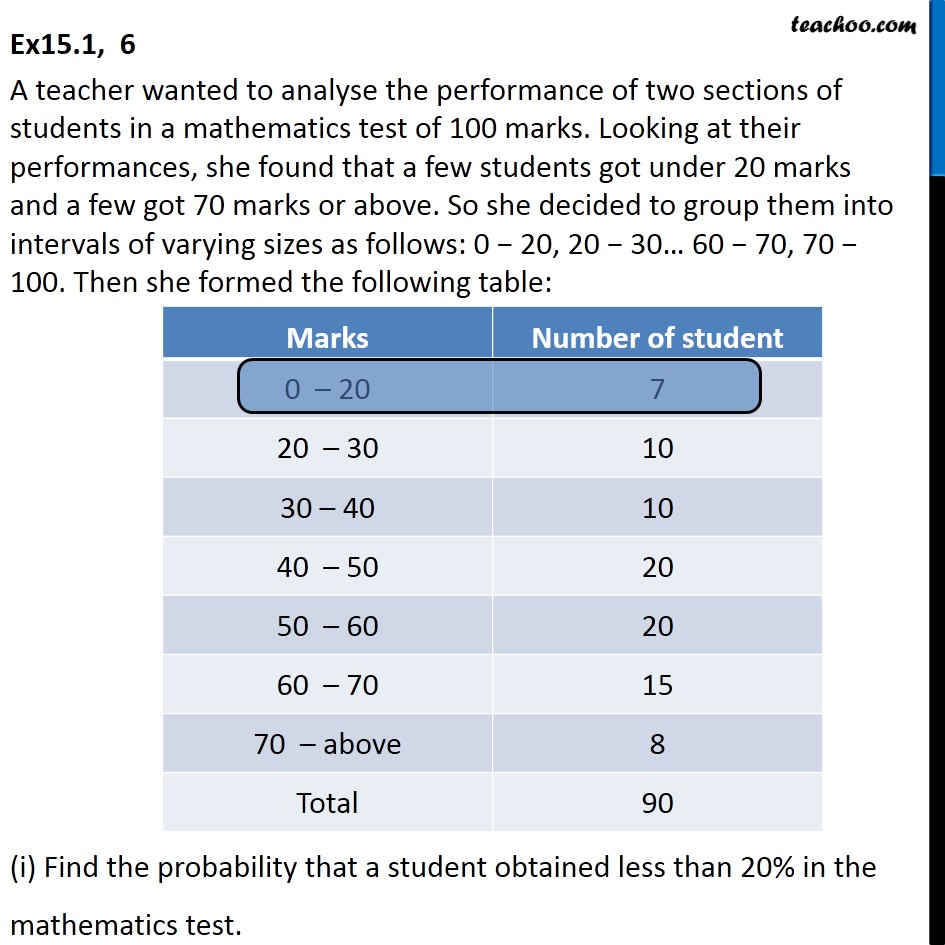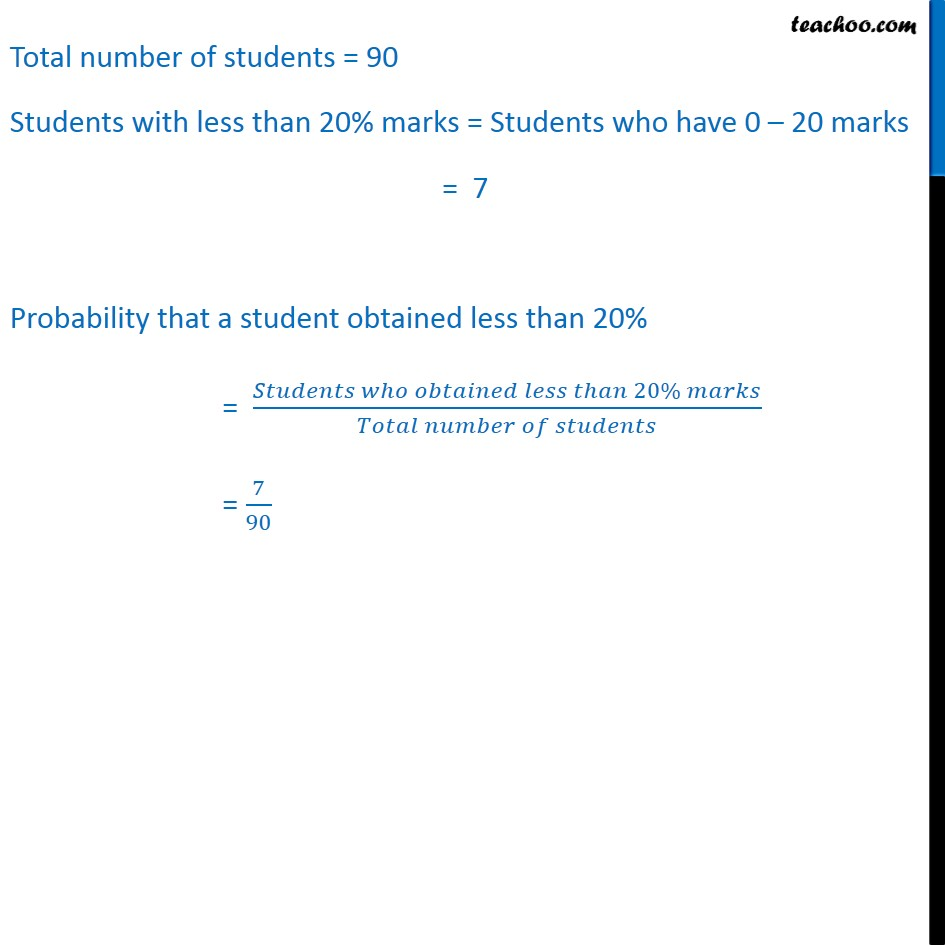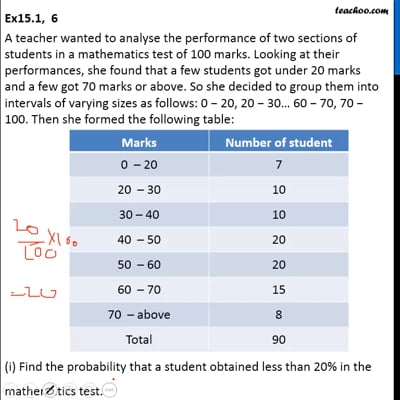This video is only available for Teachoo black users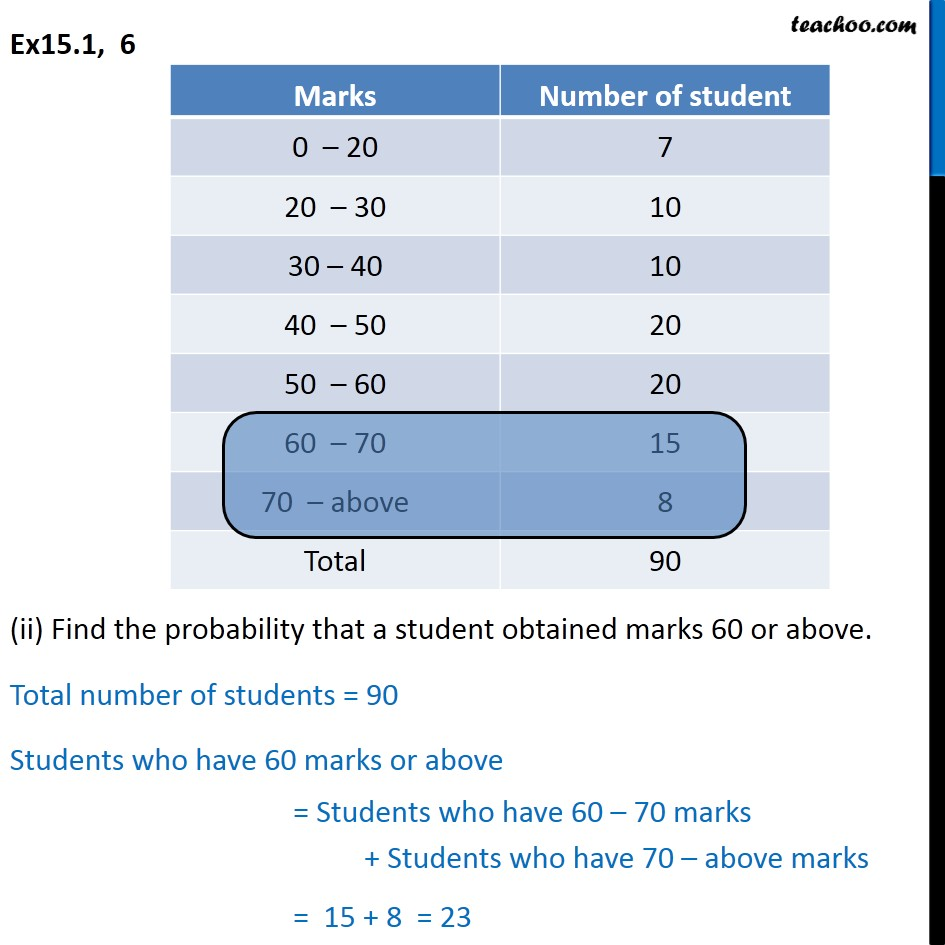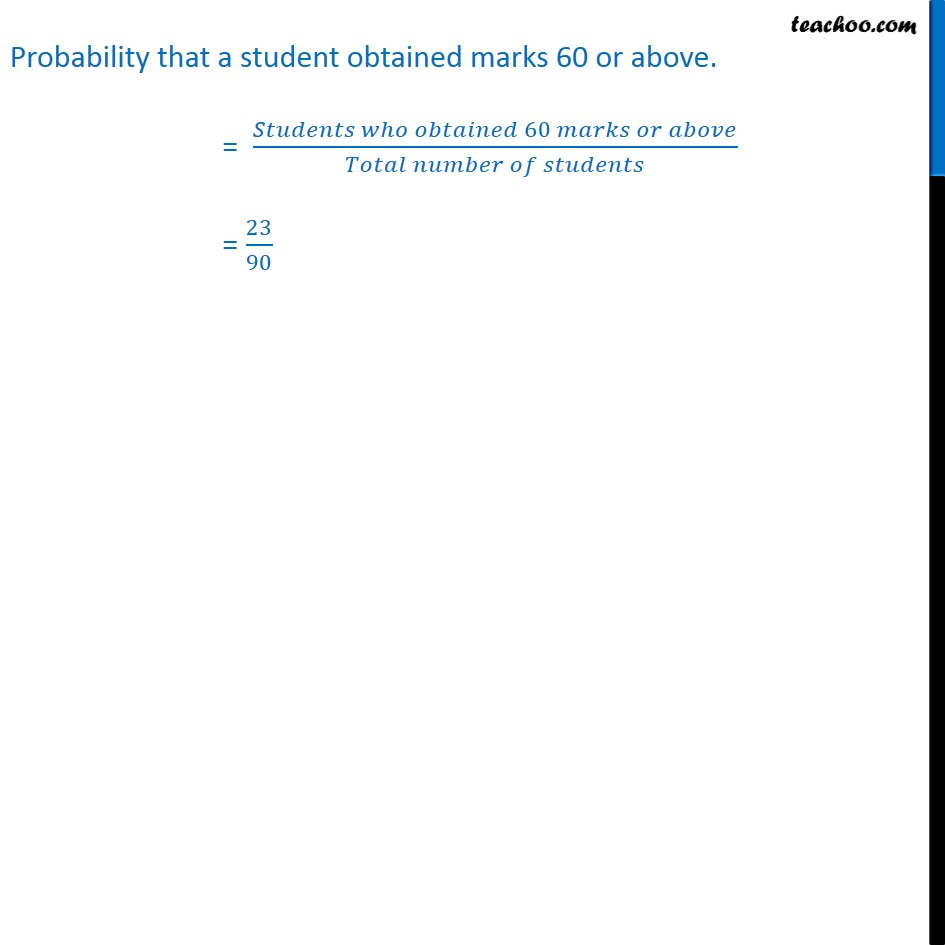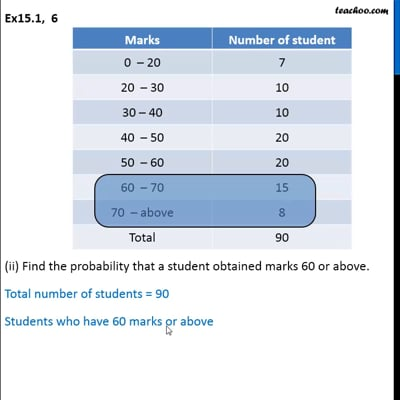This video is only available for Teachoo black users

Maths Crash Course - Live lectures + all videos + Real time Doubt solving!

### Transcript

Ex15.1, 6 A teacher wanted to analyse the performance of two sections of students in a mathematics test of 100 marks. Looking at their performances, she found that a few students got under 20 marks and a few got 70 marks or above. So she decided to group them into intervals of varying sizes as follows: 0 − 20, 20 − 30… 60 − 70, 70 − 100. Then she formed the following table: (i) Find the probability that a student obtained less than 20% in the mathematics test. Total number of students = 90 Students with less than 20% marks = Students who have 0 – 20 marks = 7 Probability that a student obtained less than 20% = (𝑆𝑡𝑢𝑑𝑒𝑛𝑡𝑠 𝑤ℎ𝑜 𝑜𝑏𝑡𝑎𝑖𝑛𝑒𝑑 𝑙𝑒𝑠𝑠 𝑡ℎ𝑎𝑛 20% 𝑚𝑎𝑟𝑘𝑠)/(𝑇𝑜𝑡𝑎𝑙 𝑛𝑢𝑚𝑏𝑒𝑟 𝑜𝑓 𝑠𝑡𝑢𝑑𝑒𝑛𝑡𝑠) = 7/90 Ex15.1, 6 (ii) Find the probability that a student obtained marks 60 or above. Total number of students = 90 Students who have 60 marks or above = Students who have 60 – 70 marks + Students who have 70 – above marks = 15 + 8 = 23 Probability that a student obtained marks 60 or above. = (𝑆𝑡𝑢𝑑𝑒𝑛𝑡𝑠 𝑤ℎ𝑜 𝑜𝑏𝑡𝑎𝑖𝑛𝑒𝑑 60 𝑚𝑎𝑟𝑘𝑠 𝑜𝑟 𝑎𝑏𝑜𝑣𝑒)/(𝑇𝑜𝑡𝑎𝑙 𝑛𝑢𝑚𝑏𝑒𝑟 𝑜𝑓 𝑠𝑡𝑢𝑑𝑒𝑛𝑡𝑠) = 23/90